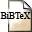# A characterization of graphs having all (g,f)-factors

## Abstract

Let G be a graph with vertex set V and let g and f be two functions assigning nonnegative integers to the vertices of G. We say that G has all (g,f)-factors, if G has an h-factor for every function h such that g(v) <= h(v) <= f(v) for every vertex v. In this note, we derive from Tutte's f-factor theorem a similar charcterization for the property of having all (g,f)-factors. An analogous result for parity-factors is presented also.

## BibTEX Reference Entry```@article{Ni98,
author = {Thomas Niessen},
title = "A characterization of graphs having all (g,f)-factors",
pages = "152--156",
journal = "Journal of Combinatorial Theory, Series B",
volume = "72",
number = "1",
doi = 10.1006/jctb.1997.1797,
year = 1998,
hsb = RWTH-CONV-223160,
}
```Download bibtex-file